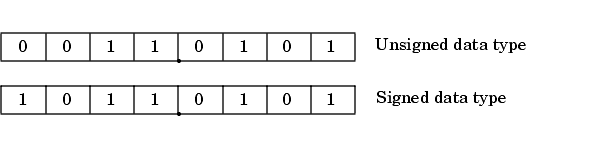Documentation

## Quantization

The quantization Q of a real-world value V is represented by a weighted sum of bits. Within the context of the general slope and bias encoding scheme, the value of an unsigned fixed-point quantity is given by

`$\stackrel{~}{V}=S.\left[\sum _{i=0}^{ws-1}{b}_{i}{2}^{i}\right]+B,$`

while the value of a signed fixed-point quantity is given by

`$\stackrel{~}{V}=S.\left[-{b}_{ws-1}{2}^{ws-1}+\sum _{i=0}^{ws-2}{b}_{i}{2}^{i}\right]+B,$`

where

• ${b}_{i}$ are binary digits, with ${b}_{i}=1,0$, for $i=0,1,...,ws-1$

• The word size in bits is given by ws, with ws = `1`, `2`, `3`,..., `128`.

• S is given by $F={2}^{E}$, where the scaling is unrestricted because the binary point does not have to be contiguous with the word.

${b}_{i}$ are called bit multipliers and ${2}^{i}$ are called the weights.

### Fixed-Point Format

Formats for 8-bit signed and unsigned fixed-point values are shown in the following figure.Note that you cannot discern whether these numbers are signed or unsigned data types merely by inspection since this information is not explicitly encoded within the word.

The binary number `0011.0101` yields the same value for the unsigned and two's complement representation because the MSB = `0`. Setting B = `0` and using the appropriate weights, bit multipliers, and scaling, the value is

`$\begin{array}{c}\stackrel{~}{V}=\left(F{2}^{E}\right)Q={2}^{E}\left[\sum _{i=0}^{ws-1}{b}_{i}{2}^{i}\right]\\ ={2}^{-4}\left(0×{2}^{7}+0×{2}^{6}+1×{2}^{5}+1×{2}^{4}+0×{2}^{3}+1×{2}^{2}+0×{2}^{1}+1×{2}^{0}\right)\\ =3.3125.\end{array}$`

Conversely, the binary number `1011.0101` yields different values for the unsigned and two's complement representation since the MSB = `1`.

Setting B = `0` and using the appropriate weights, bit multipliers, and scaling, the unsigned value is

`$\begin{array}{c}\stackrel{~}{V}=\left(F{2}^{E}\right)Q={2}^{E}\left[\sum _{i=0}^{ws-1}{b}_{i}{2}^{i}\right]\\ ={2}^{-4}\left(1×{2}^{7}+0×{2}^{6}+1×{2}^{5}+1×{2}^{4}+0×{2}^{3}+1×{2}^{2}+0×{2}^{1}+1×{2}^{0}\right)\\ =11.3125,\end{array}$`

while the two's complement value is

`$\begin{array}{c}\stackrel{~}{V}=\left(F{2}^{E}\right)Q={2}^{E}\left[-{b}_{ws-1}{2}^{ws-1}+\sum _{i=0}^{ws-2}{b}_{i}{2}^{i}\right]\\ ={2}^{-4}\left(-1×{2}^{7}+0×{2}^{6}+1×{2}^{5}+1×{2}^{4}+0×{2}^{3}+1×{2}^{2}+0×{2}^{1}+1×{2}^{0}\right)\\ =-4.6875.\end{array}$`
##### SupportGet trial now